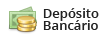# Ponteira Arrow Race-Tech - KTM 990 6'~14'

Código: 71763 Marca:
Selecione a opção de Ponteira :
6x de R\$ 1.498,50
ou R\$ 8.271,72 via Depósito
Comprar Estoque: 60 dias úteis
6x de R\$ 1.119,83
ou R\$ 6.181,48 via Depósito
Comprar Estoque: 60 dias úteis
6x de R\$ 1.119,83
ou R\$ 6.181,48 via Depósito
Comprar Estoque: 60 dias úteis
6x de R\$ 1.119,83
A partir de R\$ 6.719,00
ou R\$ 6.181,48 via Depósito
• 1x de R\$ 8.991,00 sem juros
• 2x de R\$ 4.495,50 sem juros
• 3x de R\$ 2.997,00 sem juros
• 4x de R\$ 2.247,75 sem juros
• 5x de R\$ 1.798,20 sem juros
• 6x de R\$ 1.498,50 sem juros
• 1x de R\$ 8.991,00 sem juros
• 2x de R\$ 4.495,50 sem juros
• 3x de R\$ 2.997,00 sem juros
• 4x de R\$ 2.418,24
• 5x de R\$ 1.962,66
• 6x de R\$ 1.659,16
• 7x de R\$ 1.442,56
• 8x de R\$ 1.280,28
• 9x de R\$ 1.154,21
• 10x de R\$ 1.053,48
• 11x de R\$ 971,18
• 12x de R\$ 902,71
• R\$ 8.271,72• 1x de R\$ 6.719,00 sem juros
• 2x de R\$ 3.359,50 sem juros
• 3x de R\$ 2.239,66 sem juros
• 4x de R\$ 1.679,75 sem juros
• 5x de R\$ 1.343,80 sem juros
• 6x de R\$ 1.119,83 sem juros
• 1x de R\$ 6.719,00 sem juros
• 2x de R\$ 3.359,50 sem juros
• 3x de R\$ 2.239,66 sem juros
• 4x de R\$ 1.807,16
• 5x de R\$ 1.466,70
• 6x de R\$ 1.239,89
• 7x de R\$ 1.078,03
• 8x de R\$ 956,76
• 9x de R\$ 862,54
• 10x de R\$ 787,26
• 11x de R\$ 725,77
• 12x de R\$ 674,60
• R\$ 6.181,48• 1x de R\$ 6.719,00 sem juros
• 2x de R\$ 3.359,50 sem juros
• 3x de R\$ 2.239,66 sem juros
• 4x de R\$ 1.679,75 sem juros
• 5x de R\$ 1.343,80 sem juros
• 6x de R\$ 1.119,83 sem juros
• 1x de R\$ 6.719,00 sem juros
• 2x de R\$ 3.359,50 sem juros
• 3x de R\$ 2.239,66 sem juros
• 4x de R\$ 1.807,16
• 5x de R\$ 1.466,70
• 6x de R\$ 1.239,89
• 7x de R\$ 1.078,03
• 8x de R\$ 956,76
• 9x de R\$ 862,54
• 10x de R\$ 787,26
• 11x de R\$ 725,77
• 12x de R\$ 674,60
• R\$ 6.181,48• 1x de R\$ 6.719,00 sem juros
• 2x de R\$ 3.359,50 sem juros
• 3x de R\$ 2.239,66 sem juros
• 4x de R\$ 1.679,75 sem juros
• 5x de R\$ 1.343,80 sem juros
• 6x de R\$ 1.119,83 sem juros
• 1x de R\$ 6.719,00 sem juros
• 2x de R\$ 3.359,50 sem juros
• 3x de R\$ 2.239,66 sem juros
• 4x de R\$ 1.807,16
• 5x de R\$ 1.466,70
• 6x de R\$ 1.239,89
• 7x de R\$ 1.078,03
• 8x de R\$ 956,76
• 9x de R\$ 862,54
• 10x de R\$ 787,26
• 11x de R\$ 725,77
• 12x de R\$ 674,60
• R\$ 6.181,48* Este prazo de entrega está considerando a disponibilidade do produto + prazo de entrega.